# Inverse scattering, multi-dimensional case

(diff) ← Older revision | Latest revision (diff) | Newer revision → (diff)

There are many multi-dimensional inverse scattering problems. Below, inverse potential scattering and inverse geophysical scattering are briefly discussed; see Obstacle scattering for inverse obstacle scattering problems.

## Inverse potential scattering.

To formulate the inverse potential scattering problem, consider first the direct scattering problem (see [a1], [a2], [a4], [a5], [a6], Appendix):(a1)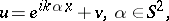(a2)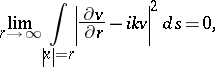(a3)

whereis given,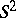is the unit sphere,is the scattered field,is the scattering solution, condition (a3) is called the (outgoing) radiation condition,is the incident plane wave, and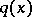is a real-valued function, called a potential,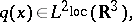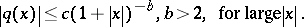The existence and uniqueness of the solution to (a1)–(a3) has been proved under less restrictive assumptions on[a2]. The functionhas the form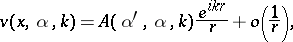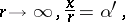where the coefficient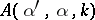is called the scattering amplitude.

The inverse potential scattering problem consists of findinggivenon some subsets of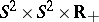.

The first result is simple: Ifis known for alland all, thenis uniquely determined.

Ifthen it is known (e.g. [a6], p. 233, see also [a4]) that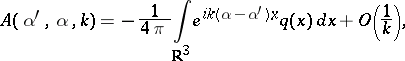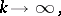so that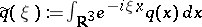can be found: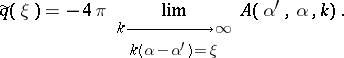The second result is much more difficult.

For decades it was not known if the data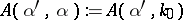,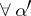,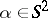and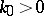fixed, determineuniquely. In 1987 the uniqueness result has been established by A.G. Ramm (see [a7], [a8]) under the assumptions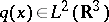,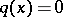for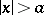, where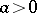is an arbitrary large fixed number, and in 1988 inversion procedures were published; see [a7]. One of them, proposed by Ramm, is based on the formula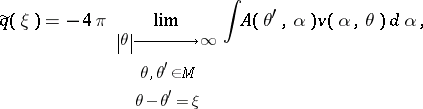where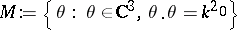,,, and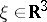is an arbitrary point.

Another inversion procedure ([a3], [a7]) is based on the reconstruction of the Dirichlet-to-Neumann mapping and then finding.

Error estimates for Ramm's inversion procedure in the case of noisy data and an algorithm for calculating the function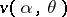in the inversion formula are obtained in [a9].

The uniqueness problem for inverse potential scattering with the data,,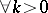,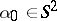, fixed, is still open (as of 2000).

The same is true for the uniqueness problem for inverse potential scattering with the (backscattering) data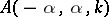,,, although for this problem a uniqueness theorem for smallholds.

## Inverse geophysical scattering.

The inverse geophysical scattering problem consists of finding the unknown coefficient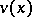in the equation(a4)

wheresatisfies the outgoing radiation condition (a3),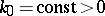is fixed, andis a real-valued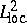function with compact support in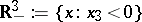.

The scattering data are the values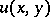,, that is, the values ofon the surface of the Earth. The functiondescribes an inhomogeneity in the velocity profile (in the refraction coefficient),can be an acoustic pressure. Uniqueness of the solution to inverse geophysical scattering problem was proved in 1987 [a8], [a7].

The uniqueness problem for inverse geophysical scattering with data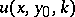,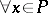,, and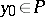fixed, is open (as of 2000).

A reduction of the inverse geophysical scattering problem with the data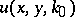,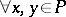, to the inverse potential scattering problem with the data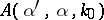,,fixed,, with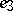the unit vector along-axis, is done in [a7].

## Inverse potential scattering: Open problem.

An interesting open problem (as of 2000) in inverse potential scattering is the problem of finding discontinuities ofand the number of bound states of the Schrödinger operator generated by the expressioninfrom the knowledge of fixed energy scattering data,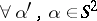.

If, then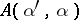is an analytic function of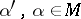. Therefore, knowledge ofon an open set in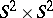, however small, allows one to recoveron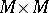.

The assumption concerning compactness of the support ofis natural in inverse potential scattering because the scattering data are always noisy and it is not possible in principle to recover the tail of a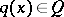(that is,for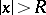, whereis sufficiently large) from knowledge of noisy data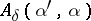,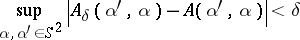(see [a7] for a proof).

How to Cite This Entry:
Inverse scattering, multi-dimensional case. Encyclopedia of Mathematics. URL: http://encyclopediaofmath.org/index.php?title=Inverse_scattering,_multi-dimensional_case&oldid=16465
This article was adapted from an original article by A.G. Ramm (originator), which appeared in Encyclopedia of Mathematics - ISBN 1402006098. See original article